Worksheet Question - The Triangles and its properties

# Worksheet Question - The Triangles and its properties - Mathematics (Maths) Class 7

Q.1. Which of the following figures will have its altitude outside the triangle.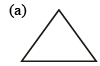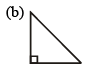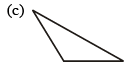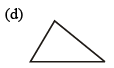Ans. C

Q.2. Fill up the blanks : (i) Every triangle has at least ................. acute angles.
(ii) The longest side of a right angled triangle is called its ...............
(iii) Median is also called .................. in an equilateral triangle.
(iv) The line segment joining a vertex of a triangle to the mid-point of its opposite side is called its.............

Ans. (i) 2
(ii) Hypotenuse
(iii) Altitude or Perpendicular bisector
(iv) Median

Q.3. If one angle of a triangle is 60° and the other two angles are in the ratio 1 : 2, then find the angles.

Ans. 40°, 80°

Q.4. In figure find the vlue of ∠A + ∠B + ∠C + ∠D + ∠E + ∠F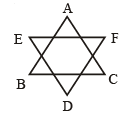Ans. 360°

Q.5. Two poles of 8m and 14m stand upright on a plane ground. If the distance between two tops is 10m.
Find the distance between their feet.

Ans. 8m

Q.6. Mohini walks 1200m due East and then 500m due North. How far is she from her starting point?

Ans. 1300 m

Q.7. Find the value of x and y. (i) Here CD || AB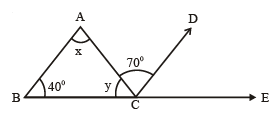(ii)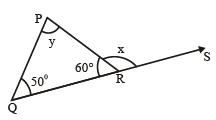(iii)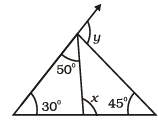(iv)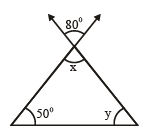Ans. (i) x = 70° y = 70°
(ii) x = 120° y = 70°
(iii) x = 80° y = 75°
(iv) x = 80° y = 50°

Q.8. Find the value of x :

(i)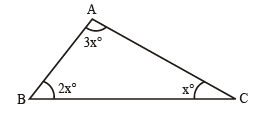(ii)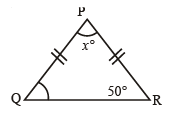(iii)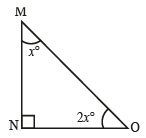(iv)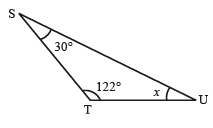Ans. (i) 30
(ii) 80°
(iii) 30
(iv) 80

Q.9. ABC is an equilateral triangle with side a. AD is an altitude. Find the value of AD2.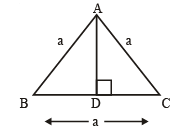Ans. AD2 =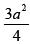Q.10. State whether the given statements are True or False :
(i) Sum of two sides of a triangle is greater than or equal to the third side.
(ii) The difference between the lengths of any two sides of a triangle is smaller than the length of third side.
(iii) Sum of any two angles of a triangle is always greater than the third angle.
(iv) It is possible to have a right angled equilateral triangle.

Ans. (i) False
(ii) True
(iii) False
(iv) False

The document Worksheet Question - The Triangles and its properties | Mathematics (Maths) Class 7 is a part of the Class 7 Course Mathematics (Maths) Class 7.
All you need of Class 7 at this link: Class 7

## Mathematics (Maths) Class 7

121 videos|351 docs|45 tests

## FAQs on Worksheet Question - The Triangles and its properties - Mathematics (Maths) Class 7

 1. What are the properties of a triangle?Ans. The properties of a triangle include having three sides, three angles, and the sum of the interior angles always equaling 180 degrees.
 2. How can I find the area of a triangle?Ans. The area of a triangle can be calculated by using the formula A = 1/2 * base * height, where the base is the length of the triangle's bottom side and the height is the perpendicular distance from the base to the opposite vertex.
 3. What are the different types of triangles based on their angles?Ans. Triangles can be classified into three types based on their angles: acute (all angles are less than 90 degrees), obtuse (one angle is greater than 90 degrees), and right (one angle is exactly 90 degrees).
 4. How can I determine if two triangles are congruent?Ans. Two triangles are congruent if their corresponding sides and angles are equal. This can be determined using congruence criteria such as side-side-side (SSS), side-angle-side (SAS), angle-side-angle (ASA), angle-angle-side (AAS), or the hypotenuse-leg (HL) criteria for right triangles.
 5. Is it possible for a triangle to have two right angles?Ans. No, it is not possible for a triangle to have two right angles. The sum of the angles in any triangle is always 180 degrees, and if two angles are already right angles (each measuring 90 degrees), the third angle would have to be 0 degrees, which is not possible in a triangle.

## Mathematics (Maths) Class 7

121 videos|351 docs|45 testsExplore Courses for Class 7 examSignup to see your scores go up within 7 days! Learn & Practice with 1000+ FREE Notes, Videos & Tests.
10M+ students study on EduRev
Track your progress, build streaks, highlight & save important lessons and more!
Related Searches

,

,

,

,

,

,

,

,

,

,

,

,

,

,

,

,

,

,

,

,

,

;